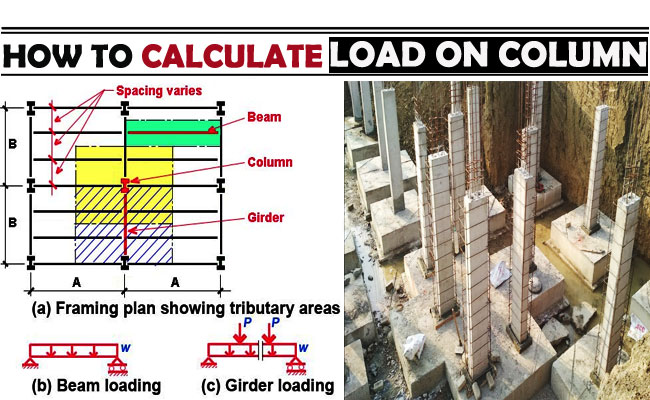### How to Calculate Load on ColumnIn this construction article, you will be familiar with the methods required for measuring the loads which operate on column, beam, wall and slab in a building.

The total weight of beam, wall and slab is borne by the column and transferred to foundation.

Given below, the details about the types of loads operating on the column:-

The self weight of column x Total number of floors

Self weight of beam per running meter x total length of beam

Load of wall per running meter x total number of meters for wall construction

Total loads operating on slab which involve dead load (generated for storing furniture & other things), live load (generated for human movement), self weight etc.

Irrespective of above mentioned loads, the bending moment also operates on column, beam, wall and slab. This should be taken into consideration in final design.

Measurement of loads on column

Self weight of concrete is 2400 kg/m3 (240 KN)

Self weight of steel is 7850 kg/m3 (8000 kg/m3 approx)

Suppose, the size of the column is 230 mm x 600 mm having 1% steel and 3 m standard height.

So, the volume of concrete = 0.600 x 0.23 x 3 = 0.414 m3 (density of concrete).

= 993.6 = 994 kg (approx)

So, the weight of steel (1%) of concrete volume

= 0.414 x 8000 mg/m3 x 1% (0.01)[density of steel = 8000 kg/m3]

So, steel = 33 kg

Therefore, total weight of column = weight of concrete + weight of steel

= 994 + 33 = 1026 kg or 10 KN for each floor

At the time of making measurement, the self weight of column is taken as 10 to 15 KN according to IS standard.

Measurement of load on beam

Suppose each meter of beam contains dimension 230 mm x 450 mm except slab.

So, volume of concrete in beam:-

= 0.230 x 0.450 x 1 = 0.138 m3

So, weight of beam per running meter should be as follow:-

= volume x density of concrete

= 0.138 x 2400 Kg/m3 = 333 kg

So, the weight of concrete inside beam is 333 kg.

Assume, the quantity of steel in beam as 2% (as per guidelines provided in the book by B.N. Dutta)

So, the weight of steel will be calculated as follow:-

Volume of concrete x density of steel x percentage of steel

= 0.138 x 8000 x 0.02 = 22 kg

So, total load of beam per running meter will be as follow:-

Weight of concrete + weight of steel = 333 + 22 = 355 kg/m = 3.5 KN/m

Load calculation on slab

Here, self weight of the slab should be considered for one square meter

Suppose, the density of slab = 125 mm

The weight of the slab will be as follow:-

Volume x density = 0.125 x 1m2 x 2400 kg/m3 = 300 kg = 3 KN

The load for floor finish is taken as 1 KN per meter and live load is taken as 2 KN per meter. Therefore, from the measurement provided in above, the slab load should be 6 to 7 KN per square meter.

Calculation of load for wall

Density of brick generally remains at 1500 to 2000 Kg/m3

Here, the dimension of brick is taken as follow:-

Thickness of brick wall = 6 inch

Height of brick wall = 3 m

Length of brick wall = 1 m

Load of brick masonry work per running meter is as follow:-

= thickness x length x height x density = 0.150 x 1 x 3 x 2000 = 900 kg = 9 KN/m2 (as 6” = 150 mm = 0.150 m)

For aerated or autoclaved concrete block, the load of the slab should be 550 kg to 700 kg/meter

When, the block is used, the load of the wall is taken as 4 KN/meter (according to IS: 456)

Factor of safety should be considered as 1.5 according to IS: 456: 2000